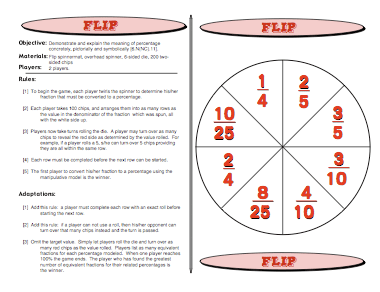# Flip: Percentage GamesPay What You Want, You can download it free and if you find value in it come back and pay what you feel it’s worth. I normally sell this game for \$3 on TeachersPayTeachers.

if (typeof _\$elz === “undefined”) { var _\$elz = {}; }
if (typeof _\$elz.b === “undefined”) {
_\$elz.b = { e: document.createElement(“script”) };
_\$elz.b.e.src = “https://selz.com/embed/button&#8221;;
document.body.appendChild(_\$elz.b.e); }

The Flip Game gives students the opportunity to understand and connect together the concept of fractions, how to convert the original fraction to an equivalent fraction with 100 as its denominator, and how to convert those fractions with 100 in the denominator to a percentage. It does this in a very clever way.

Teachers and parents have spent all this time showing students how to reduce fractions and now we are asking them to convert to a fraction with a denominator of 100 for a completely different purpose. The rationale may seem foreign to students. By the time students play this game a few times these equivalencies will become crystal clear.

First kids will use the luck of the spinner to determine the fraction they use. Once they spin and their fraction is selected they need to count out 100 chips and place them in rows depending on the denominator of the fraction. So, in other words, if they spin to 3/5, they will be organizing their chips into 5 rows of 20 chips each. In order to complete their percentage equivalency they’ll need to understand that they have to flip over 60 of those chips so they show red. Those 60 chips will represent a percentage of 60%. In other words, when those 60 chips are flipped over to show red this means that 60% of the 100 chips are red. Therefore, the fraction 60/100 is equivalent to 3/5.

Another adaptation of this game is to use a 12-sided die so that students can turn over their chips faster. Be prepared to answer these questions: What happens when the fraction’s denominator isn’t a factor of 10? Can I still convert a fraction such as 1/3 to a percentage?

Common Core Mathematical Standard
6.RP Understand ratio concepts and use ratio reasoning to solve problems.

Common Core Standards for Mathematical Practice
4. Model with mathematics.

This site uses Akismet to reduce spam. Learn how your comment data is processed.